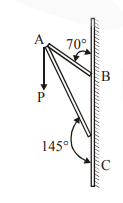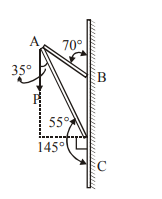# Consider a frame that is made up of two thin massless rods AB and AC as shown in the figure.`
Question:

Consider a frame that is made up of two thin massless rods AB and AC as shown in the figure. A vertical force G P of magnitude 100 N is applied at point A of the frameSuppose the force is G P resolved parallel to the arms AB and AC of the frame. The magnitude of the resolved component along the arm AC is xN. The value of x, to the nearest integer, is________

$\left[\right.$ Given : $\sin \left(35^{\circ}\right)=0.573, \cos \left(35^{\circ}\right)=0.819$

$\left.\sin \left(110^{\circ}\right)=0.939, \cos \left(110^{\circ}\right)=-0.342\right]$

Solution:Component along AC

$=100 \cos 35^{\circ} \mathrm{N}$

$=100 \times 0.819 \mathrm{~N}$

$=81.9 \mathrm{~N}$

$\approx 82 \mathrm{~N}$